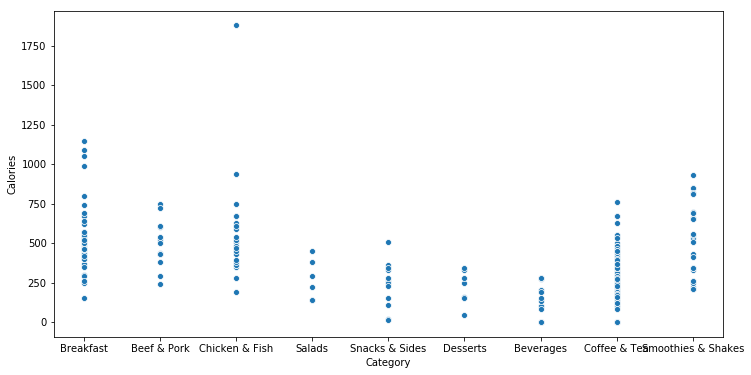# Correlation and Two-Variable Relationships

Last updated: January 13th, 2020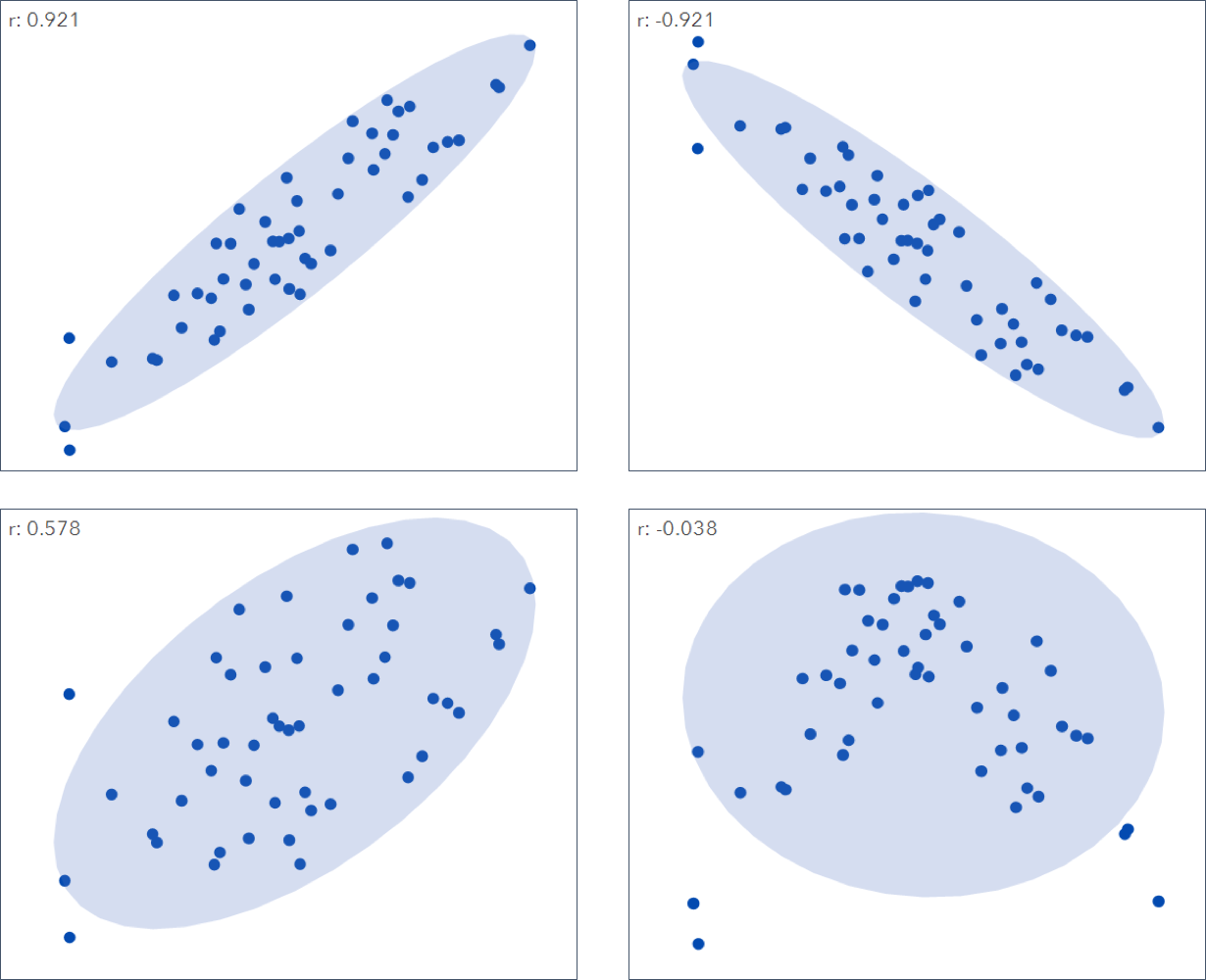# Correlation and two-variable relationships¶

Exploratory data analysis in many modeling projects, whether in data science or in research, involves examining correlation among predictors, and between predictors and a target variable.

Also, the standard way to visualize the relationship between two measured data variables is with scatter plots with on variable on each X and Y axis.## Hands on!¶

In :
import numpy as np
import pandas as pd
import matplotlib.pyplot as plt
import seaborn as sns

%matplotlib inline


We'll use the following McDonald's menu nutrition facts dataset in this lesson.

This dataset provides a nutrition analysis of every menu item on the US McDonald's menu, including breakfast, beef burgers, chicken and fish sandwiches, fries, salads, soda, coffee and tea, milkshakes, and desserts.

In :
df = pd.read_csv('data/mcdonalds_menu.csv')


Out:
Category Item Serving Size Calories Calories from Fat Total Fat Total Fat (% Daily Value) Saturated Fat Saturated Fat (% Daily Value) Trans Fat ... Carbohydrates Carbohydrates (% Daily Value) Dietary Fiber Dietary Fiber (% Daily Value) Sugars Protein Vitamin A (% Daily Value) Vitamin C (% Daily Value) Calcium (% Daily Value) Iron (% Daily Value)
0 Breakfast Egg McMuffin 4.8 oz (136 g) 300 120 13.0 20 5.0 25 0.0 ... 31 10 4 17 3 17 10 0 25 15
1 Breakfast Egg White Delight 4.8 oz (135 g) 250 70 8.0 12 3.0 15 0.0 ... 30 10 4 17 3 18 6 0 25 8
2 Breakfast Sausage McMuffin 3.9 oz (111 g) 370 200 23.0 35 8.0 42 0.0 ... 29 10 4 17 2 14 8 0 25 10
3 Breakfast Sausage McMuffin with Egg 5.7 oz (161 g) 450 250 28.0 43 10.0 52 0.0 ... 30 10 4 17 2 21 15 0 30 15
4 Breakfast Sausage McMuffin with Egg Whites 5.7 oz (161 g) 400 210 23.0 35 8.0 42 0.0 ... 30 10 4 17 2 21 6 0 25 10

5 rows × 24 columns### Correlation¶

Correlation is one of the most widely used statistical concepts.

The correlation coefficient is a metric that measures the extent to which numeric variables are associated with one another. It takes values from -1 to +1.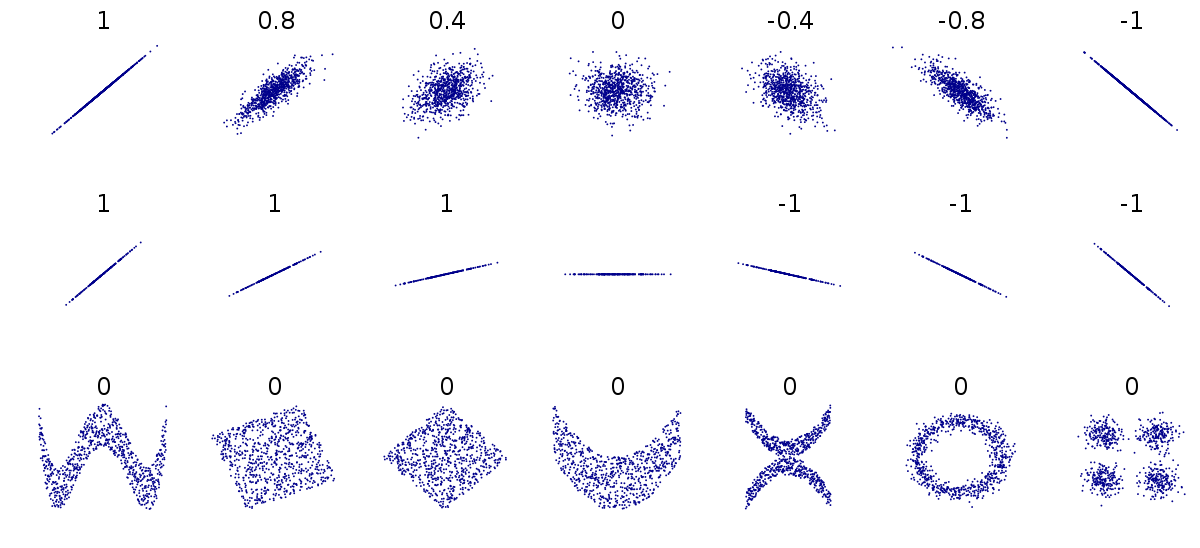The correlation matrix is a table where the variables are shown on both rows and columns, and the cell values are the correlations between the variables.

In :
df.dtypes

Out:
Category                          object
Item                              object
Serving Size                      object
Calories                           int64
Calories from Fat                  int64
Total Fat                        float64
Total Fat (% Daily Value)          int64
Saturated Fat                    float64
Saturated Fat (% Daily Value)      int64
Trans Fat                        float64
Cholesterol                        int64
Cholesterol (% Daily Value)        int64
Sodium                             int64
Sodium (% Daily Value)             int64
Carbohydrates                      int64
Carbohydrates (% Daily Value)      int64
Dietary Fiber                      int64
Dietary Fiber (% Daily Value)      int64
Sugars                             int64
Protein                            int64
Vitamin A (% Daily Value)          int64
Vitamin C (% Daily Value)          int64
Calcium (% Daily Value)            int64
Iron (% Daily Value)               int64
dtype: object
In :
corr = df.corr()

sns.heatmap(corr)

Out:
<matplotlib.axes._subplots.AxesSubplot at 0x7fd225bd7470>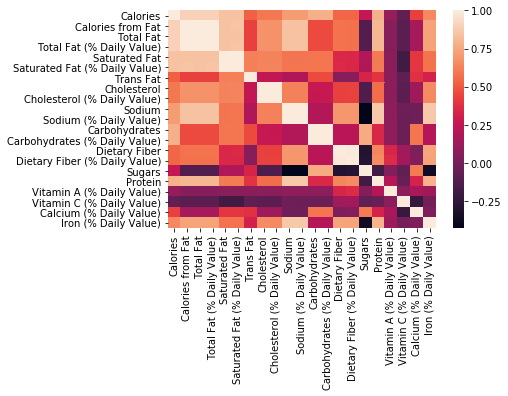The seaborn's heatmap function accepts many parameters to make our plot looks prettier:

In :
# Generate a mask for the upper triangle

plt.figure(figsize=(18, 10))

center=0, square=True, annot=True, fmt=".1f")

Out:
<matplotlib.axes._subplots.AxesSubplot at 0x7fd223aa7d68>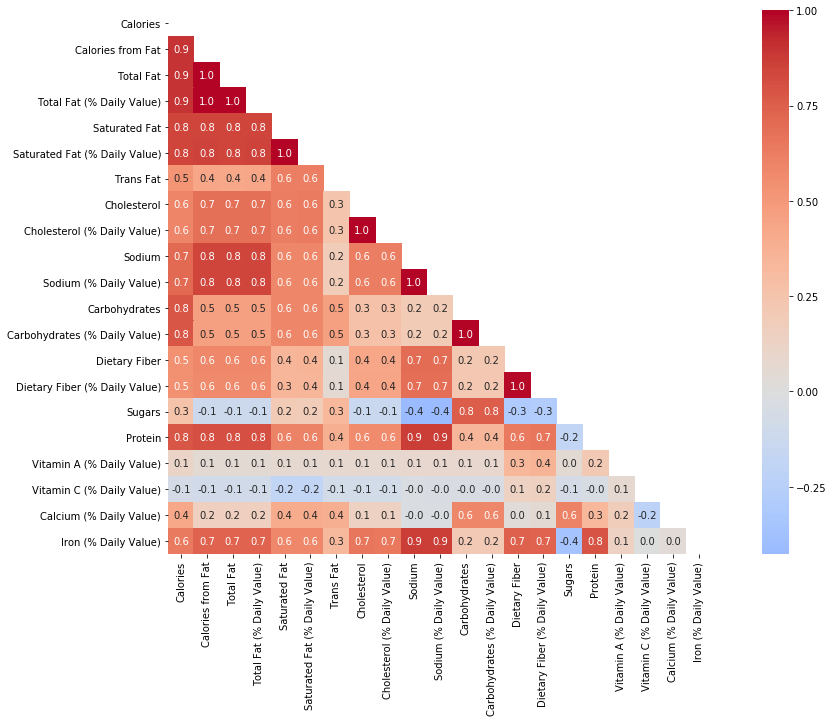### Two-variable relationships¶

A scatterplot is a plot in which the x-axis is the value of one variable, and the y-axis the value of another.

#### Numeric - Numeric¶

In :
fig, axs = plt.subplots(1, 4, figsize=(18, 4))

cols = ['Sodium', 'Carbohydrates', 'Total Fat', 'Calcium (% Daily Value)']

for i, col in enumerate(cols):
sns.scatterplot(x='Cholesterol', y=col, data=df, size=1, legend=False, ax=axs[i])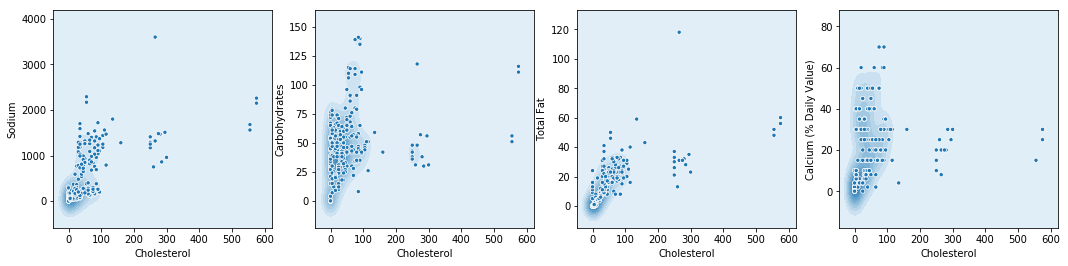In :
plt.figure(figsize=(12, 6))

sns.scatterplot(x='Carbohydrates', y='Sodium', data=df, size=1, legend=False)

Out:
<matplotlib.axes._subplots.AxesSubplot at 0x7fd2238e4e48>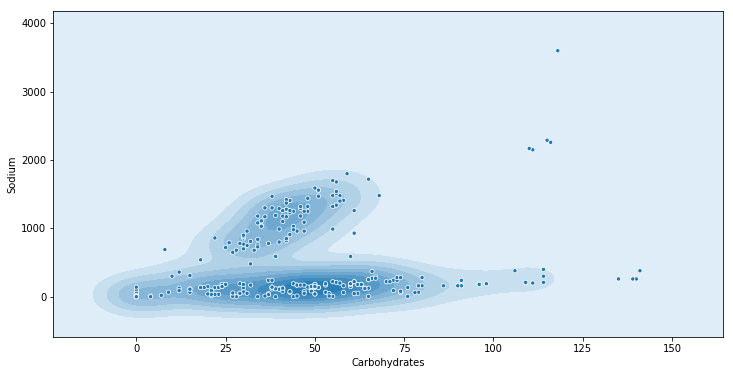In :
plt.figure(figsize=(12, 6))

sns.scatterplot(x='Carbohydrates', y='Cholesterol', data=df, size=1, legend=False)

Out:
<matplotlib.axes._subplots.AxesSubplot at 0x7fd2237b4908>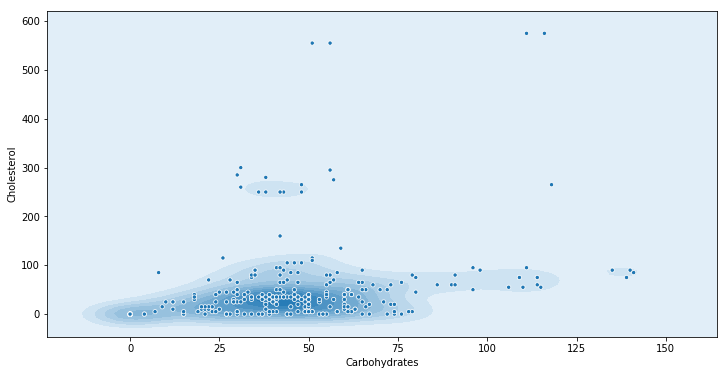In :
sns.pairplot(data=df, vars=['Calories', 'Total Fat', 'Sugars', 'Protein'])

Out:
<seaborn.axisgrid.PairGrid at 0x7fd223894588>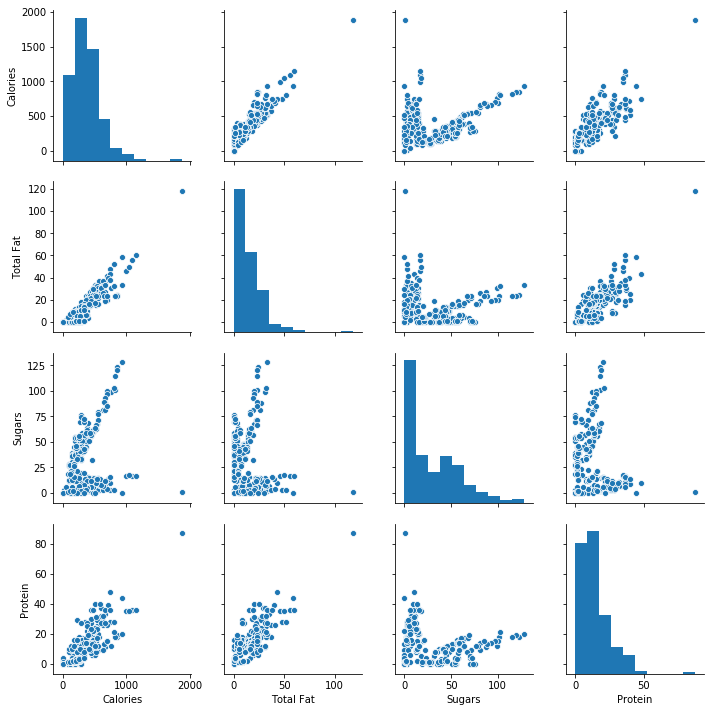In :
plt.figure(figsize=(12, 6))

sns.scatterplot(x='Sugars', y='Protein', data=df)

Out:
<matplotlib.axes._subplots.AxesSubplot at 0x7fd2216bfd68>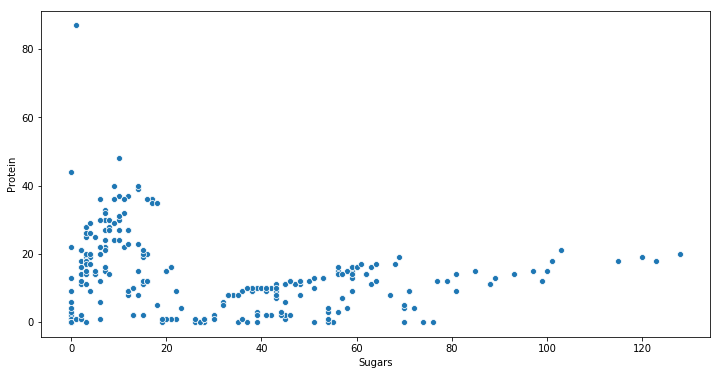In :
plt.figure(figsize=(14, 6))

sns.regplot(x='Sugars', y='Protein', data=df)

Out:
<matplotlib.axes._subplots.AxesSubplot at 0x7fd221815978>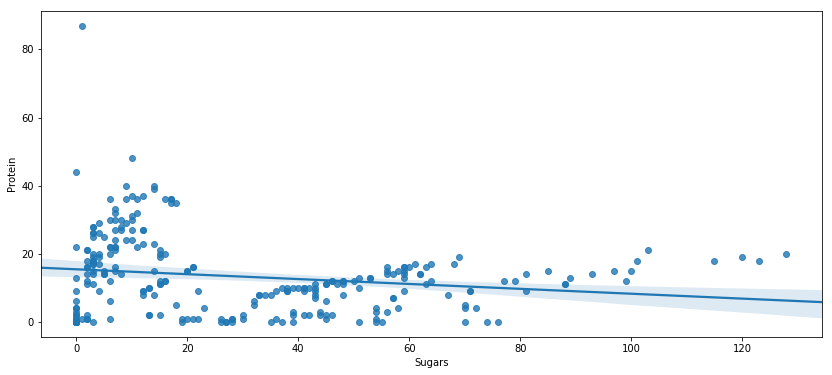#### Numeric - Categorical¶

In :
plt.figure(figsize=(12, 6))

sns.boxplot(x='Category', y='Calories', data=df)

Out:
<matplotlib.axes._subplots.AxesSubplot at 0x7fd223845390>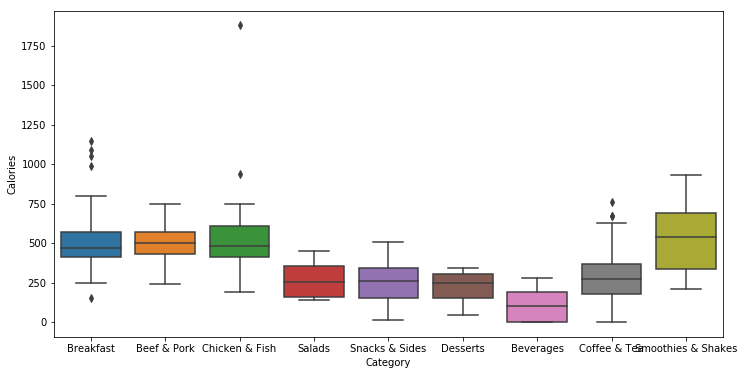In :
plt.figure(figsize=(12, 6))

sns.scatterplot(x='Category', y='Calories', data=df)

Out:
<matplotlib.axes._subplots.AxesSubplot at 0x7fd2213c9978>